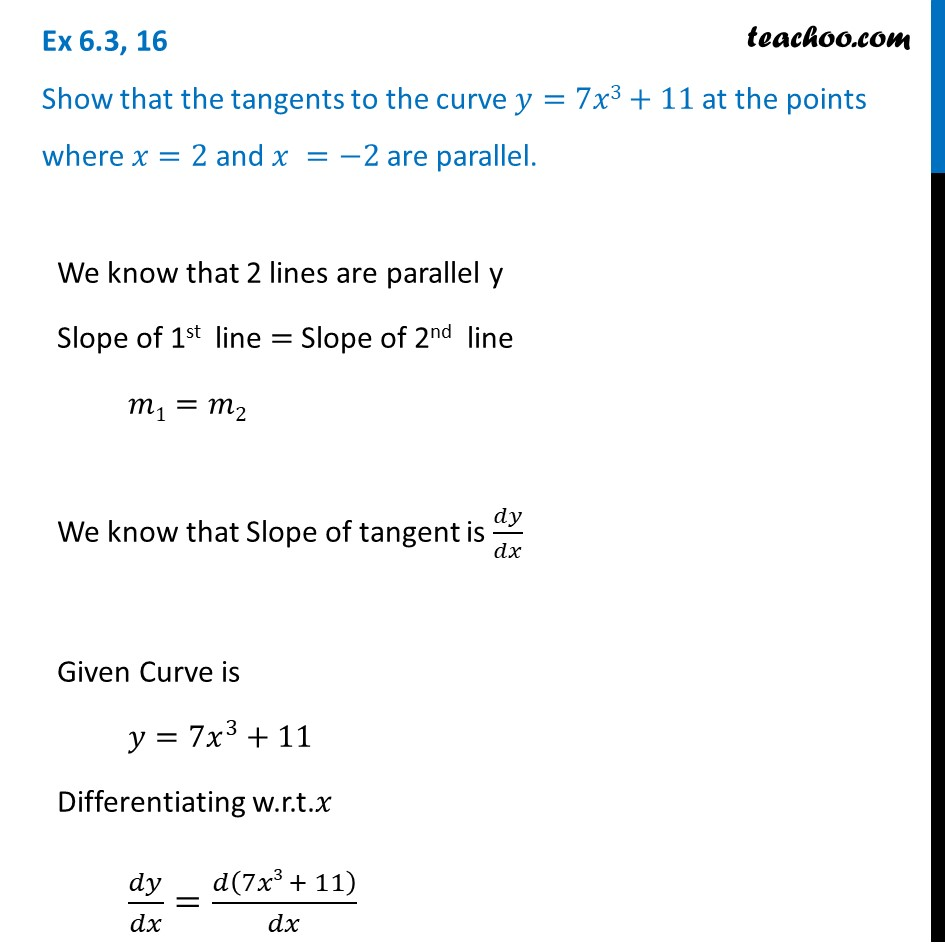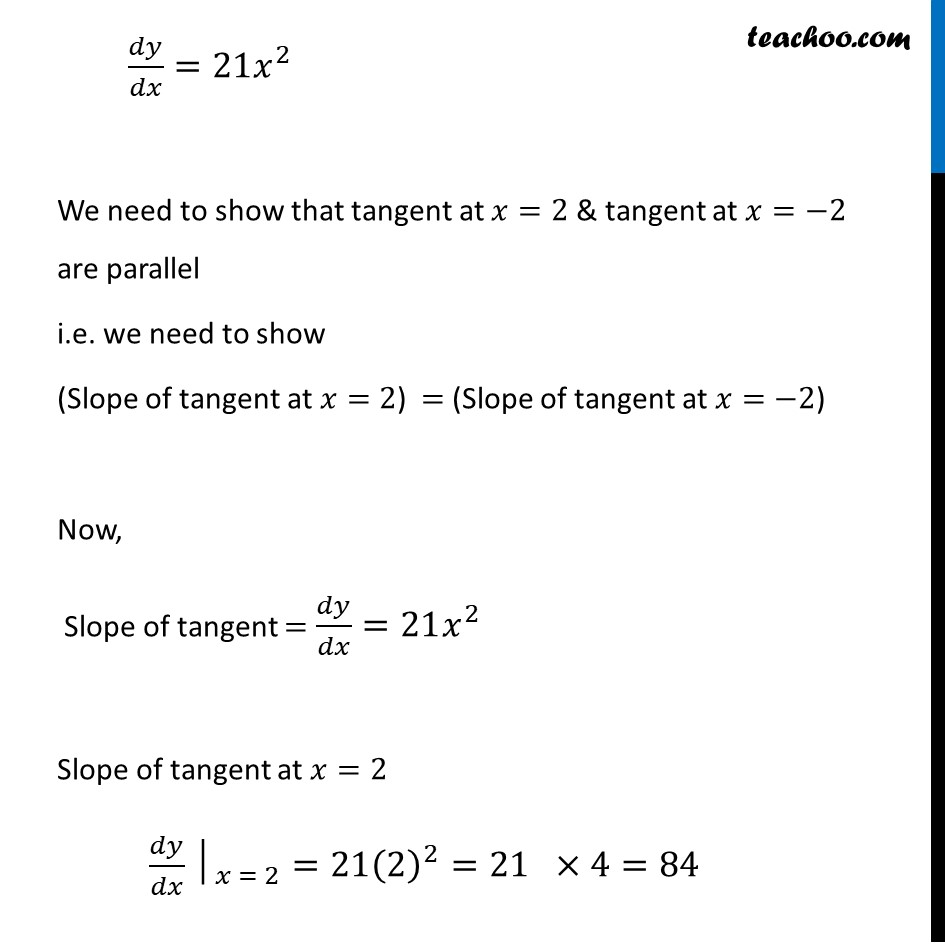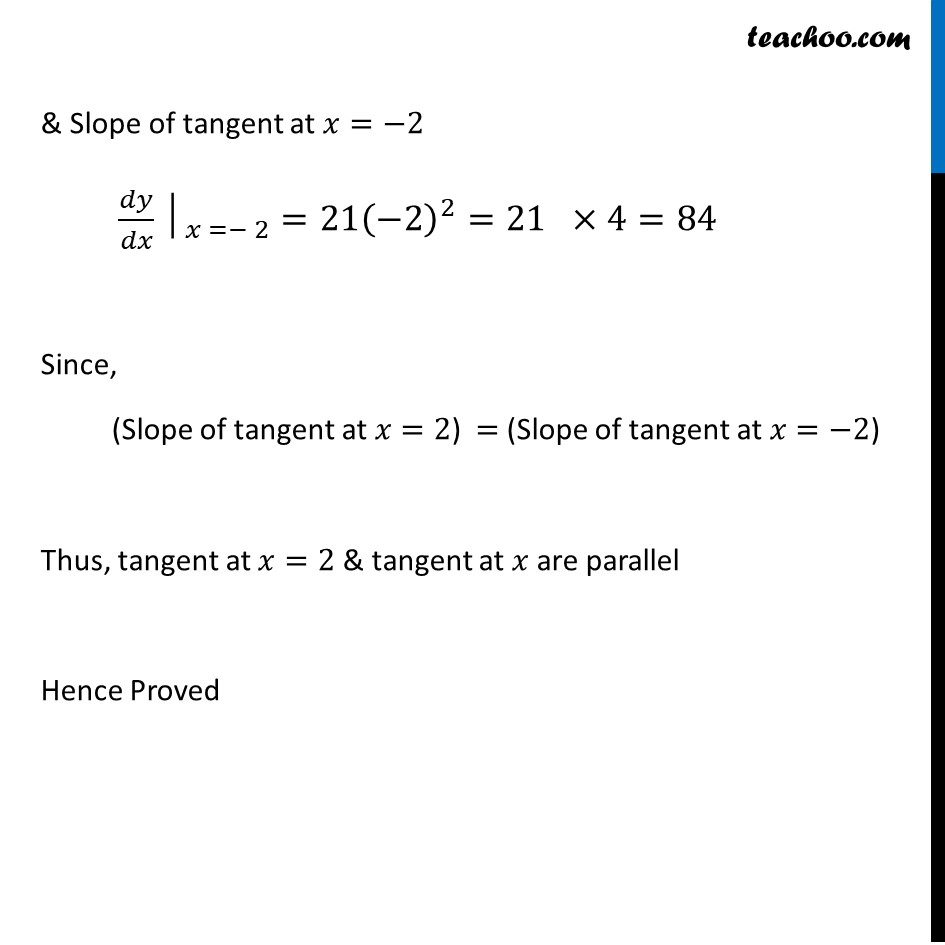Finding point when tangent is parallel/ perpendicular

Chapter 6 Class 12 Application of Derivatives
Concept wiseIntroducing your new favourite teacher - Teachoo Black, at only ₹83 per month

### Transcript

Ex 6.3, 16 Show that the tangents to the curve 𝑦=7𝑥3+11 at the points where 𝑥=2 and 𝑥 =−2 are parallel.We know that 2 lines are parallel y Slope of 1st line = Slope of 2nd line 𝑚1=𝑚2 We know that Slope of tangent is 𝑑𝑦/𝑑𝑥 Given Curve is 𝑦=7𝑥^3+11 Differentiating w.r.t.𝑥 𝑑𝑦/𝑑𝑥=𝑑(7𝑥3 + 11)/𝑑𝑥 𝑑𝑦/𝑑𝑥=21𝑥^2 We need to show that tangent at 𝑥=2 & tangent at 𝑥=−2 are parallel i.e. we need to show (Slope of tangent at 𝑥=2) = (Slope of tangent at 𝑥=−2) Now, Slope of tangent = 𝑑𝑦/𝑑𝑥=21𝑥^2 Slope of tangent at 𝑥=2 〖𝑑𝑦/𝑑𝑥│〗_(𝑥 = 2)=21(2)^2=21 ×4=84 & Slope of tangent at 𝑥=−2 〖𝑑𝑦/𝑑𝑥│〗_(𝑥 =− 2)=21(−2)^2=21 ×4=84 Since, (Slope of tangent at 𝑥=2) = (Slope of tangent at 𝑥=−2) Thus, tangent at 𝑥=2 & tangent at 𝑥 are parallel Hence Proved